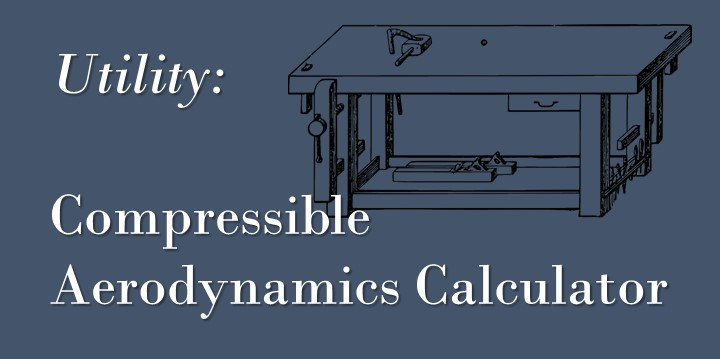Skip to content# Tagoblique shock calculator## Compressible Aerodynamics Calculator – Matlab

Here I have written a Matlab App for calculating compressible aerodynamics using the basic gas dynamics equations. The calculations available are: Isentropic Flow (including Prandtl-Meyer flow) Normal Shocks (See article) … Continue Reading Compressible Aerodynamics Calculator – Matlab